# MATCH_RECOGNIZE and predicates - everything you need to know

Senior Principal Product Manager## MATCH_RECOGNIZE and predicates

At a recent user conference I had a question about when and how  predicates are applied when using MATCH_RECOGNIZE so that’s the purpose of this blog post. Will this post cover everything you will ever need to know for this topic? Probably!

Where to start….the first thing to remember is that the table listed in the FROM clause of your SELECT statement acts as the input into the MATCH_RECOGNIZE pattern matching process and this raises the question about how and where are predicates actually applied. I briefly touched on this topic in part 1 of my deep dive series on MATCH_RECOGNIZE: SQL Pattern Matching Deep Dive - Part 1.

In that first post I looked at the position of predicates within the explain plan and their impact on sorting. In this post I am going to use the built in measures (MATCH_NUMBER and CLASSIFIER) to show the impact of applying predicates to the results that are returned.

First, if you need a quick refresher course in how to use the MATCH_RECOGNIZE built-in measures then see part 2 of the deep dive series: SQL Pattern Matching Deep Dive - Part 2, using MATCH_NUMBER() and CLASSIFIER().

As per usual I am going to use my normal stock ticker schema to illustrate the specific points. You can find this schema listed on most of the pattern matching examples on livesql.oracle.com. There are three key areas within the MATCH_RECOGNIZE clause that impact on predicates…

1. PARTITION BY column
2. ORDER BY column
3. All other columns

### 1. Predicates on the PARTITION BY column

```select * from ticker
MATCH_RECOGNIZE(
PARTITION BY symbol ORDER BY tstamp
MEASURES match_number() as mn
ALL ROWS PER MATCH
PATTERN (strt)
DEFINE strt as 1=1
);```

Note that we are using an always-true pattern STRT which is defined as 1=1 to ensure that we process all rows and the pattern has no range so it will be matched once and then reset to find the next match. As our ticker table contains 60 rows, the output also contains 60 rows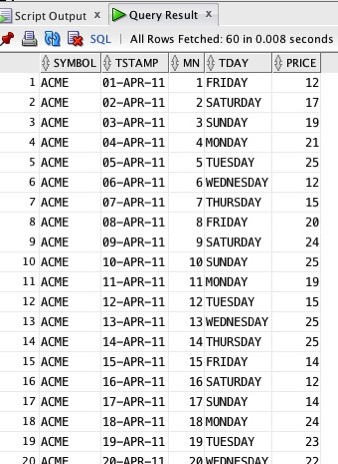Checkout the column headed mn which contains our match_numnber() measure. This shows that within the first partition for ACME we matched the always-true event 20 times, i.e. all rows were matched. If we check the explain plan for this query we can see that all 60 rows (3 symbols, and 20 rows for each symbol) were processed: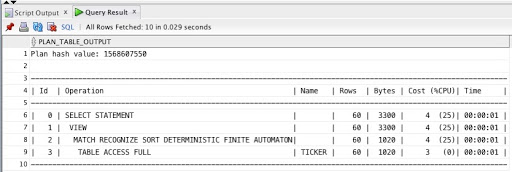If we now apply a predicate on the PARTITION BY column, SYMBOL, then we can see that the first “block” of our output looks exactly the same, however, the explain plan shows that we have processed fewer rows - only 20 rows.

Let’ modify and rerun our simple query:

```select * from ticker
MATCH_RECOGNIZE(
PARTITION BY symbol ORDER BY tstamp
MEASURES match_number() as mn
ALL ROWS PER MATCH
PATTERN (strt+)
DEFINE strt as 1=1
)
WHERE symbol = ‘ACME';```

the results look similar but note that the output summary returned by SQL Developer indicates that only 20 rows were fetched: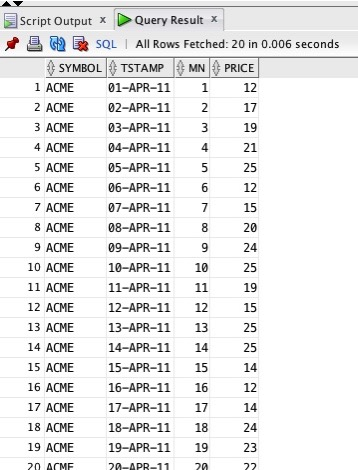notice that the match_number() column (mn) is showing 1 - 20 as values returned from the pattern matching process. If we look at the explain plan….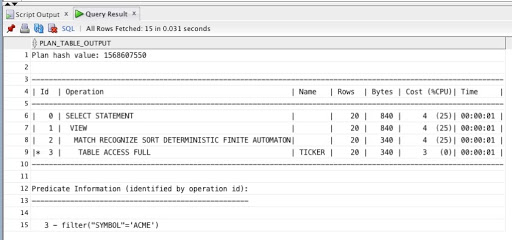…this also shows that we processed 20 rows - so partition elimination filtered out the other 40 rows before pattern matching started. Therefore, if you apply predicates on the PARTITION BY column then MATCH_RECOGNIZE is smart enough to perform partition elimination to reduce the number of rows that need to be processed.

### Conclusion - predicates on the PARTITION BY column.

Predicates on the partition by column reduce the amount of data being passed into MATCH_RECOGNIZE.

Built-in measures such as MATCH_NUMBER work as expected in that a contiguous sequence is returned.

### 2. Predicates on the ORDER BY column

What happens if we apply a predicate to the ORDER BY column? Let’s amend the query and add a filter on the tstamp column:

```select * from ticker
MATCH_RECOGNIZE(
PARTITION BY symbol ORDER BY tstamp
MEASURES match_number() as mn
ALL ROWS PER MATCH
PATTERN (strt)
DEFINE strt as 1=1
)
WHERE symbol='ACME'
AND tstamp BETWEEN '01-APR-11' AND '10-APR-11';```

returns a smaller resultset of only 10 rows and match_number is correctly sequenced from 1-10 - as expected: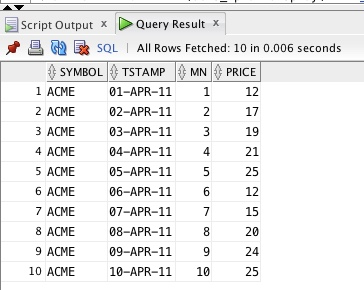however, the explain plan shows that we processed all the rows within the partition (20).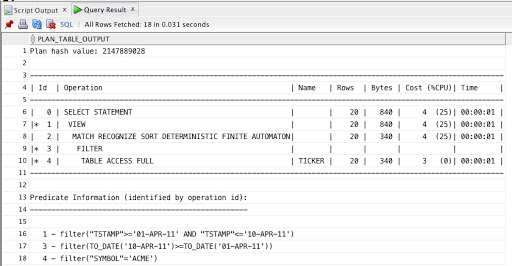This becomes a little clearer if remove the predicate on the SYMBOL column:

```select * from ticker
MATCH_RECOGNIZE(
PARTITION BY symbol ORDER BY tstamp
MEASURES match_number() as mn
ALL ROWS PER MATCH
PATTERN (strt)
DEFINE strt as 1=1
)
WHERE tstamp BETWEEN ’01-APR-11' AND '10-APR-11';```

now we see that 30 rows are returned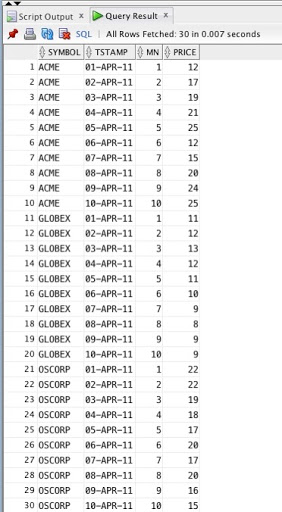but all 60 rows have actually been processed!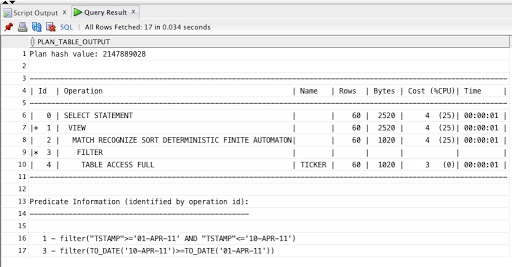### Conclusion

Filters applied to non-partition by columns are applied after the pattern matching process has completed: rows are passed in to MATCH_RECOGNIZE, the pattern is matched and then predicates on the ORDER BY/other columns are applied.

Is there a way to prove that this is actually what is happening?

### 3.Using other columns

Lets add another column to our ticker table that shows the day name for each trade. Now let’s rerun the query with the predicate on the SYMBOL column:

```select * from ticker
MATCH_RECOGNIZE(
PARTITION BY symbol ORDER BY tstamp
MEASURES match_number() as mn
ALL ROWS PER MATCH
PATTERN (strt)
DEFINE strt as 1=1
)
WHERE symbol = ‘ACME';```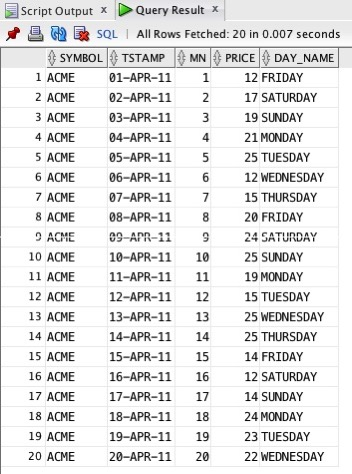the column to note is MN which contains a contiguous sequence of numbers from 1 to 20.

What happens if we filter on the day_name column and only keep the working-week days (Mon-Fri):

```select * from ticker
MATCH_RECOGNIZE(
PARTITION BY symbol ORDER BY tstamp
MEASURES match_number() as mn
ALL ROWS PER MATCH
PATTERN (strt)
DEFINE strt as 1=1
)
WHERE symbol = ‘ACME'
AND day_name in (‘MONDAY’, ’TUESDAY’, ‘WEDNESDAY’, ’THURSDAY’, ‘FRIDAY’);```

now if we look at the match_number column, mn, we can see that the sequence is no longer contiguous: the value in row 2 is now 4 and not 2, row 7 the value of mn is 11 even though the previous row was 8: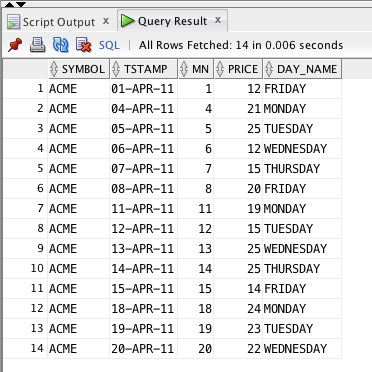It is still possible to “access” the rows that have been removed. Consider the following query with the measure PREV(day_name):

```select * from ticker
MATCH_RECOGNIZE(
PARTITION BY symbol ORDER BY tstamp
MEASURES match_number() as mn,
prev(day_name) as prev_day
ALL ROWS PER MATCH
PATTERN (strt)
DEFINE strt as 1=1
)
WHERE symbol='ACME'
AND day_name in ('MONDAY', 'WEDNESDAY', 'FRIDAY');```

this returns the following: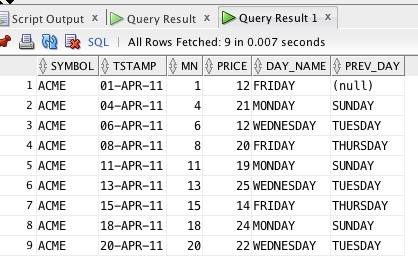where you can see that on row 2 the value for SUNDAY has been returned even though logically looking at the results the previous day should be FRIDAY.

This has important implications for numerical calculations such as running totals, final totals, averages, counts, min and max etc etc because these will take into account all the matches (depending on how your pattern is defined) prior to the final set of predicates (i.e. non-PARTITION BY columns) being applied.

## One last example

Let’s now change the always-true pattern to search for as many rows as possible (turn it into a greedy quantifier)

```select symbol, tstamp, mn, price, day_name, prev_day, total_rows from ticker
MATCH_RECOGNIZE(
PARTITION BY symbol ORDER BY tstamp
MEASURES match_number() as mn,
prev(day_name) as prev_day,
count(*) as total_rows
ALL ROWS PER MATCH
PATTERN (strt+)
DEFINE strt as 1=1
)
WHERE symbol='ACME'
AND day_name in ('MONDAY', 'WEDNESDAY', 'FRIDAY');```

the results from the following two queries:

Query 1:

```select symbol, tstamp, mn, price, day_name, prev_day, total_rows, avg_price, max_price

from ticker
MATCH_RECOGNIZE(
PARTITION BY symbol ORDER BY tstamp
MEASURES match_number() as mn,
prev(day_name) as prev_day,
count(*) as total_rows,
trunc(avg(price),2) as avg_price,
max(price) as max_price
ALL ROWS PER MATCH
PATTERN (strt+)
DEFINE strt as 1=1
)
WHERE symbol=‘ACME';```

Query 2:

```select symbol, tstamp, mn, price, day_name, prev_day, total_rows, avg_price, max_price from ticker
MATCH_RECOGNIZE(
PARTITION BY symbol ORDER BY tstamp
MEASURES match_number() as mn,
prev(day_name) as prev_day,
count(*) as total_rows,
trunc(avg(price),2) as avg_price,
max(price) as max_price
ALL ROWS PER MATCH
PATTERN (strt+)
DEFINE strt as 1=1
)
WHERE symbol='ACME'
AND day_name in ('MONDAY', 'WEDNESDAY', 'FRIDAY');```

the number of rows returned is different but the values for the calculated columns (previous day, count, max and min) are exactly the same:

Resultset 1: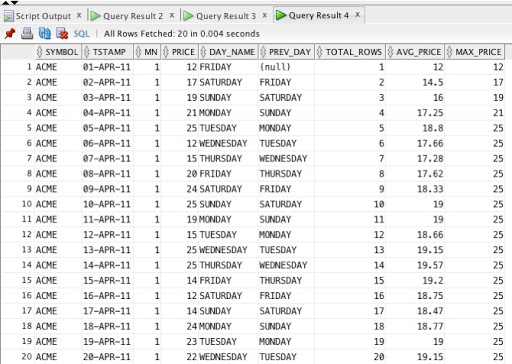Resultset 2: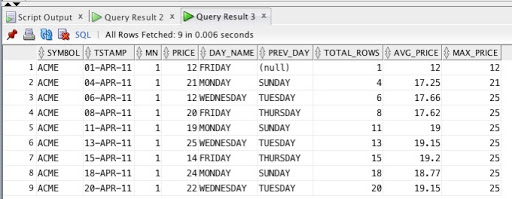## Conclusion

When I briefly touched on this topic in part 1 of my deep dive series on MATCH_RECOGNIZE, SQL Pattern Matching Deep Dive - Part 1, the focus was on the impact predicates had on sorting - would additional sorting take place if predicates were used.

In this post I have looked at the impact on the data returned. Obviously by removing rows at the end of processing there can be a huge impact on calculated measures such as match_number, counts and averages etc.

Hope this has been helpful. If you have any questions then feel free to send me an email: keith.laker@oracle.com.

Main image courtesy of wikipedia